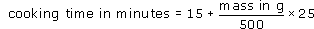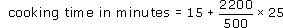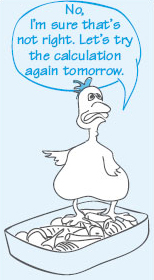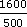Starting with maths: Patterns and formulas

Start this free course now. Just create an account and sign in. Enrol and complete the course for a free statement of participation or digital badge if available.

Free course

# 3.2 Maths in cookery

The time taken to cook a fresh chicken depends on its mass, as given by the following formula:Roughly how long will a chicken with a mass of 2.2 kg take to cook?

To use the formula, you need to substitute the mass of the chicken into the right-hand side of the equation and then work out the resulting calculation. However, the formula asks for the mass in grams, so the first step is to convert 2.2 kg into g.

Since there are 1000 g in 1 kg, 2.2 kg = 2.2 × 1000 g = 2200 g.

Substituting 2200 for the mass in g gives:Carrying out the multiplication and division first gives:

cooking time in minutes = 15 + 110 = 125.

So the cooking time would be about 125 minutes or 2 hours and 5 minutes.

If you prefer, you can use your calculator, but remember to make an estimate first so that you can check that your answer is reasonable.Figure 9

## Activity 4: A smaller bird

How long would it take to cook a chicken with a mass of 1.6 kg?

### Discussion

Converting 1.6 kg into g: 1.6 kg = 1.6 × 1000 g = 1600 g.

So the cooking time in minutes minutes = 15 +× 25 = 95.

The chicken would take about 95 minutes or 1 hour 35 mins.